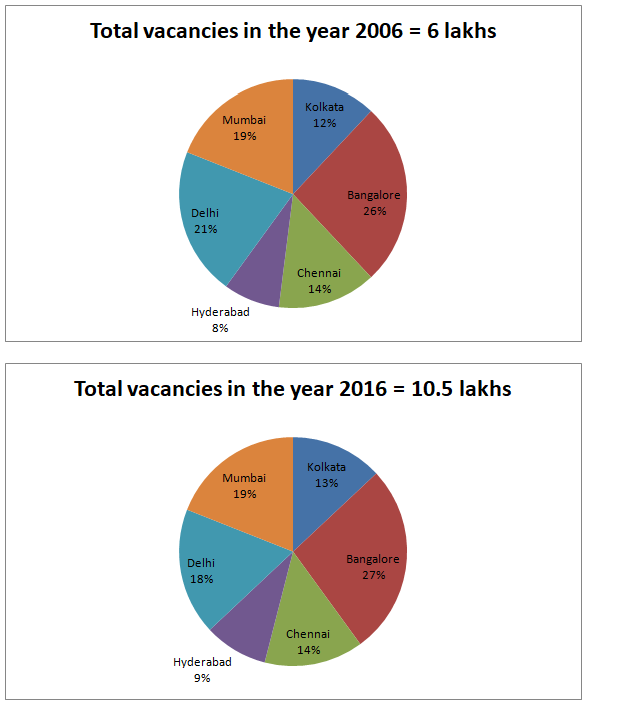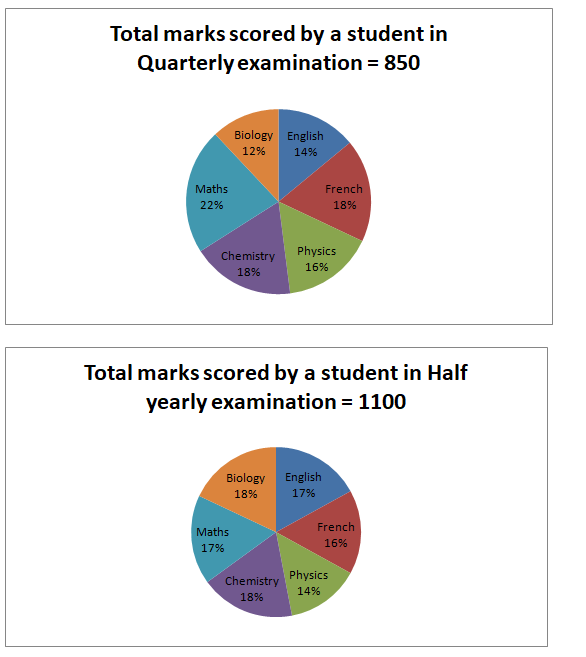# Quantitative Aptitude Questions (Data Interpretation) for SBI Clerk/PO Prelims 2018 Day-148

Dear Readers, SBI is conducting Online Examination for the recruitment of Clerical Cadre and probationary officer. To enrich your preparation here we have providing new series of Data Interpretation – Quantitative Aptitude Questions. Candidates those who are appearing in SBI Clerk/PO Prelims Exams can practice these Quantitative Aptitude average questions daily and make your preparation effective.

[WpProQuiz 2655]

Click “Start Quiz” to attend these Questions and view Solutions

Directions (Q. 1 – 5) Study the following information carefully and answer the given questions:

Following pie chart shows the percentage distribution of total vacancies in IT industries in the year 2006 and 2016 in different cities.1. Total number of vacancies in Kolkata, Chennai and Delhi together in the year 2016 is approximately what percentage more than the total number of vacancies in the same cities in the year 2006?
1. 100 %
2. 115 %
3. 90 %
4. 68 %
5. 80 %
1. If the total number of vacancies in the year 2017 is increased by 18 % while comparing with the previous year and because of recession the total number of vacancies in the year 2007 is decreased by 12 % while comparing with the previous year, then find the total number of vacancies in Bangalore in the year 2007 and Chennai in the year 2017?(Use the percentage distribution of 2006 for 2007 and that of 2016 for 2017)
1. 310740
2. 335620
3. 289560
4. 291570
5. None of these
1. Find the difference between total number of vacancies in the year 2006 in Mumbai, Chennai and Kolkata together to that of total number of vacancies in the year 2016 in Bangalore, Hyderabad and Delhi together?
1. 257800
2. 276300
3. 297000
4. 315600
5. 338000
1. If the total number of vacancies in 2010 is increased by 29 % while comparing with the year 2006, then find the total number of vacancies in 2010?
1. 668900
2. 774000
3. 712000
4. 689200
5. None of these
1. Find the ratio between the total number of vacancies in the year 2006 in Kolkata, Chennai and Delhi together to that of 2016 in the same cities together?
1. 245 : 479
2. 297 : 486
3. 193 : 212
4. 188 : 315
5. None of these

Directions (Q. 6 – 10) Study the following information carefully and answer the given questions:

Following pie chart shows the percentage distribution of marks scored by a student in different subjects in Quarterly and Half yearly examination 2017-2018.

Maximum marks in the examination is 1200. (Maximum mark- Each 200 marks, Total subjects – 6)1. Find the difference between the total marks scored by a student in Physics, French and Maths in Quarterly examination to that of English, Chemistry and Biology in Half yearly examination?
1. 145
2. 128
3. 107
4. 133
5. None of these
1. Find the ratio between the total marks scored by a student in English, French and Biology in Quarterly examination to that of total marks scored by a student in Physics, Chemistry and Maths in Half yearly examination?
1. 374 : 539
2. 356 : 493
3. 278 : 319
4. 244 : 357
5. None of these
1. Find the sum of total marks scored by a student in English, Physics and Maths in Quarterly examination and the total marks scored by a student in Biology, Chemistry and French in Half yearly examination?
1. 996
2. 912
3. 878
4. 1014
5. None of these
1. Total marks scored by a student in French, Physics and Chemistry together in Quarterly examination is approximately what percentage of total marks scored by a student in English, maths and Biology in Half yearly examination?
1. 84 %
2. 77 %
3. 95 %
4. 110 %
5. 115 %
1. What is the approximate percentage of marks scored by a student in the subject of maths, physics and chemistry in Quarterly examination while comparing with the maximum marks of these subjects?
1. 70 %
2. 85 %
3. 80 %
4. 75 %
5. 65 %

Total number of vacancies in Kolkata, Chennai and Delhi together in the year 2016

= > 10.5*(13 + 14 + 18)/100 = 4.725 lakhs

Total number of vacancies in Kolkata, Chennai and Delhi together in the year 2006

= > 6*(12 + 14 + 21)/100 = 2.82 lakhs

Required % = {(4.725 – 2.82)/2.82}*100 = 67.55 % = 68 %

The total number of vacancies in the year 2017

= > 10.5*(118/100) = 12.39 lakhs

The total number of vacancies in the year 2007

= > 6*(88/100) = 5.28 lakhs

The total number of vacancies in Bangalore in the year 2007

= > 5.28*(26/100) = 1.3728

The total number of vacancies in Chennai in the year 2017

= > 12.39*(14/100) = 1.7346

Required sum = 1.3728 + 1.7346 = 3.1074 lakhs = 310740

Total number of vacancies in the year 2006 in Mumbai, Chennai and Kolkata together

= > 6*(45/100) = 2.7 lakhs

Total number of vacancies in the year 2016 in Bangalore, Hyderabad and Delhi together

= > 10.5*(54/100) = 5.67 lakhs

Required difference = 5.67 – 2.7 = 2.97 lakhs = 297000

The total number of vacancies in 2010

= > 6*(129/100) = 7.74 lakhs

= > 774000

The total number of vacancies in the year 2006 in Kolkata, Chennai and Delhi together

= > 600000*(47/100)

The total number of vacancies in the year 2016 in Kolkata, Chennai and Delhi together

= > 1050000*(45/100)

Required ratio = [600000*(47/100)] : [1050000*(45/100)]

= > 188 : 315

Directions (Q. 6 – 10)

The total marks scored by a student in Physics, French and Maths in Quarterly examination

= > 850*(56/100) = 476

The total marks scored by a student in English, Chemistry and Biology in Half yearly examination

= > 1100*(53/100) = 583

Required difference = 583 – 476 = 107

The total marks scored by a student in English, French and Biology in Quarterly examination

= > 850*(14 + 18 + 12)/100

= > 850*(44/100) = 374

The total marks scored by a student in Physics, Chemistry and Maths in Half yearly examination

= > 1100*(14 + 18 + 17)/100

= > 1100*(49/100) = 539

Required ratio = 374 : 539

Total marks scored by a student in English, Physics and Maths in Quarterly examination

= > 850*(14 + 16 + 22)/100

= > 850*(52/100) = 442

Total marks scored by a student in Biology, Chemistry and French in Half yearly examination

= > 1100*(18 + 18 + 16)/100

= > 1100*(52/100) = 572

Required sum = 442 + 572 = 1014

Total marks scored by a student in French, Physics and Chemistry together in Quarterly examination

= > 850*(18 + 16 + 18)/100

= > 850*(52/100) = 442

Total marks scored by a student in English, maths and Biology in Half yearly examination

= > 1100*(17 + 17 + 18)/100

= > 1100*(52/100) = 572

Required % = (442/572)*100 = 77.27 % = 77 %

Total marks scored by a student in the subject of maths, physics and chemistry in Quarterly examination

= > 850*(22 + 16 + 18)/100

= > 850*(56/100) = 476

Required % = (476/600)*100 = 79.33 % = 80 %

Daily Practice Test Schedule | Good Luck

 Topic Daily Publishing Time Daily News Papers & Editorials 8.00 AM Current Affairs Quiz 9.00 AM Quantitative Aptitude “20-20” 11.00 AM Vocabulary (Based on The Hindu) 12.00 PM General Awareness “20-20” 1.00 PM English Language “20-20” 2.00 PM Reasoning Puzzles & Seating 4.00 PM Daily Current Affairs Updates 5.00 PM Data Interpretation / Application Sums (Topic Wise) 6.00 PM Reasoning Ability “20-20” 7.00 PM English Language (New Pattern Questions) 8.00 PM